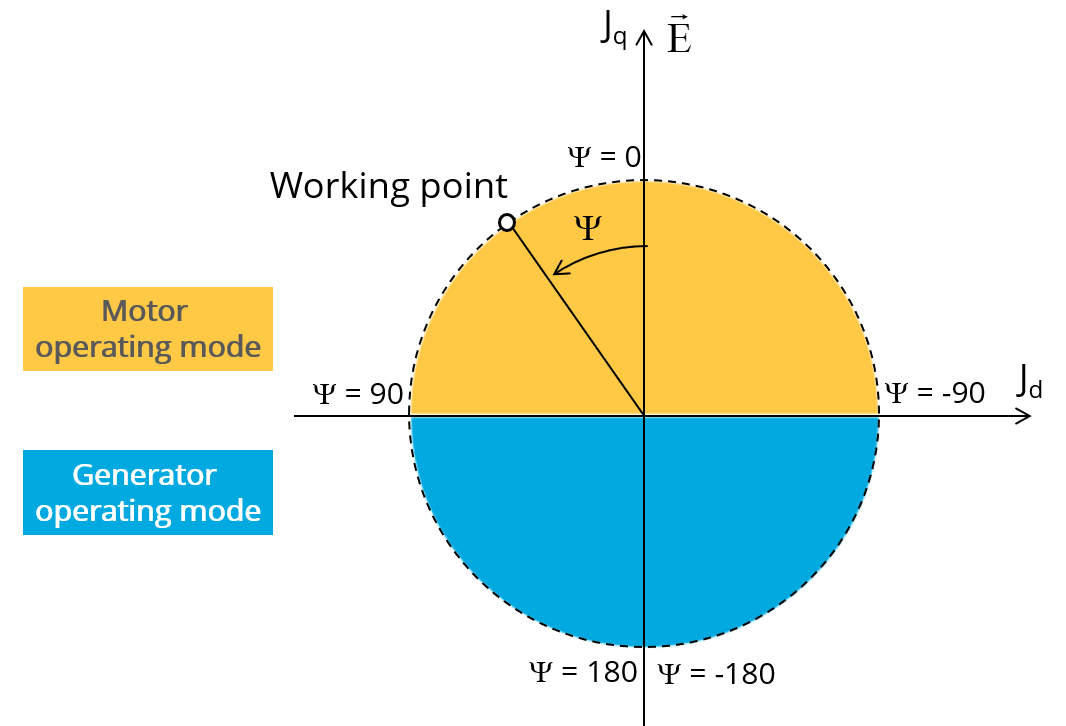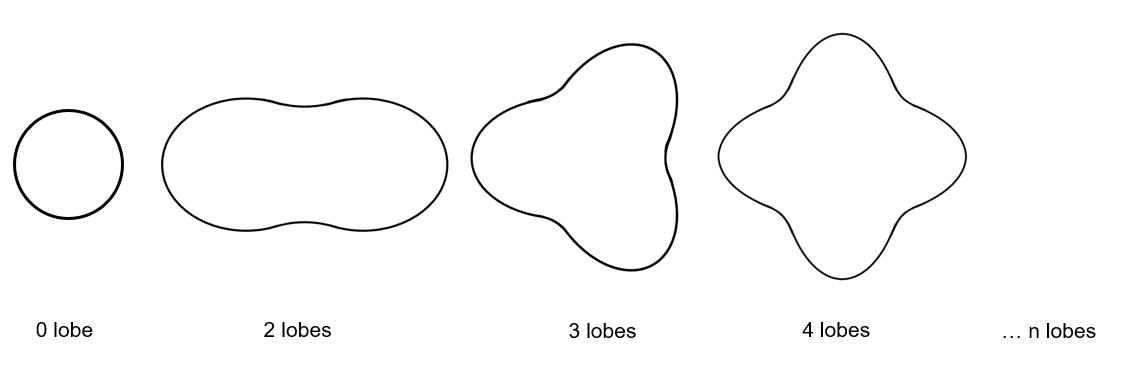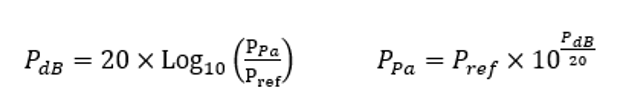# Inputs

## 1. Introduction

The total number of inputs is equal to 10.

Among these inputs, 3 are standard inputs and 7 are advanced inputs.

## 2.1 Line current / Current density

There are two common ways to define the electrical current.

Electrical current can be defined either by the current density in electric conductors or directly by indicating the value of the line current (the rms value is required).

When the choice of current definition mode is “Current”, the rms value of the line current supplied to the machine: “ Line current, rms” ( Line current, rms value ) must be provided.

When the choice of current definition mode is “Density”, the rms value of the current density in electric conductors “ Current density, rms” (Current density in conductors, rms value) must be provided.

Note: The number of parallel paths and the winding connection are automatically considered in the results.

## 2.2 Control angle

Considering the vector diagram shown below, the “ Control angle ” is the angle between the electromotive force E and the electrical current (J) (Ψ = (E, J)).

The default value is 45 degrees. It is an electrical angle. It must be set in a range of -90 to 90 degrees.

This range of values covers all the possible working point in motor convention.Definition of the control angle Ψ - Motor convention

## 2.3 Speed

The imposed “ Speed ” ( Speed ) of the machine must be set.

## 3.1 Maximum engine order / No. Points per electrical period

Two kinds of inputs are possible:

Define the Max. engine order ( Maximum engine order ) or the No. points / elec. Period ( Number of points per electric period ).

When decomposing the Maxwell pressure, applied on the stator, to get its harmonic contributions, the “ max. engine order ” ( Maximum engine order ) is required to compute its decomposition in function of the time.

At a practical point of view, when the maximum engine order is equal to N, that leads to consider 2*N computation points over a complete rotation period of the rotor.

Note:
1. The input "Engine order" is in connection with the frequency of vibration.

"Engine order" refers to a mechanical revolution period of the motor whereas frequency refers to the considered electrical period.

Obviously, both are linked with speed.

For instance, radiated sound power can be displayed either by considering frequency or engine order.

2. There are two possibilities, either set an engine order or a number of points per electrical period.

For transient computations the minimum needed number of points per electrical period is 40.

So, when the engine order is not high enough to reach this constraint It is automatically modified to get 40 computation points per electrical period.

## 3.2 Maximum mode / spatial order

The “ max. mode / spatial order ” ( Maximum mode / spatial order ) input allows the user to define the number of modes to be considered for the acoustic structural analysis. If the user selects 25, it means that the highest number of lobes in the stator deformation will be equal to 25 lobes. All deformations corresponding to more than 25 lobes will be dismissed.Number of lobes of the stator mechanical structure

## 3.3 Number of computations per tooth pitch

The “ No. comp./tooth pitch ” ( Number of computations per tooth pitch ) allows to choose the number of Maxwell pressure evaluations per tooth. The more points selected, the more accurate the Maxwell pressure harmonic decomposition will be.

## 3.4 Displayed pressure range (dB)

The “ displayed pressure range (dB) ” ( Displayed Maxwell pressure range ) is only related to the displaying of results.

It allows to increase/decrease the range of non-zero contributions displayed in the Maxwell pressure harmonic decomposition map.

When one chooses a displayed pressure range equal to 50 dB, only the contributions within the range of 50 dB and below the maximum computed value is displayed.

Note: The absolute reference value P ref (expressed in Pa), which corresponds to 0 dB, is indicated in the working point table.

Considering this referent value P ref , one can compute any Maxwell pressure value P Pa (expressed in Pa) from the ones expressed in Decibel P dB .

The relations between these quantities are illustrated below:The default value is equal to 80. The range of possible values is [20;100].

## 3.5 Computation of forces on teeth

The “ computation of forces on teeth ” ( Additional analysis to compute the force on teeth ) allows to perform or not, the additional analysis which is the computation of force on teeth. Since this computation requires bigger memory and an additional computation time, this input allows to consider it as an additional option.

## 3.6 Mesh order

To get the results, the original computation is performed using a Finite Element Modeling.

Two levels of meshing can be considered for this finite element calculation: first order and second order.

This parameter influences the accuracy of results and the computation time.

By default, second order mesh is used.

## 3.7 Airgap mesh coefficient

The advanced user input “ Airgap mesh coefficient ” is a coefficient which adjusts the size of mesh elements inside the airgap. When the value of “ Airgap mesh coefficient ” decreases, the mesh elements get smaller, leading to a higher mesh density inside the airgap, increasing the computation accuracy.

The imposed Mesh Point (size of mesh elements touching points of the geometry) is described with the following parameters:

MeshPoint = (airgap) x (airgap mesh coefficient)

Airgap mesh coefficient is set to 1.5 by default.

The variation range of values for this parameter is [0.05; 2].

0.05 giving a very high mesh density and 2 giving a very coarse mesh density.

CAUTION:
Be aware, a very high mesh density does not always mean a better result quality.

However, this always leads to a huge number of nodes in the corresponding finite element model. So, it means a need of huge numerical memory and increases the computation time considerably.